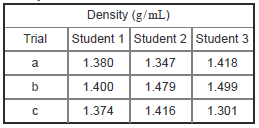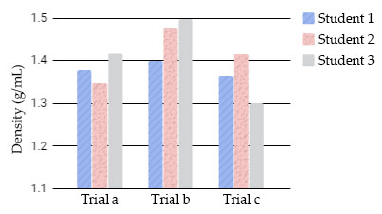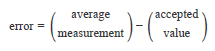# Problem: Part B. The accepted density of the sugar solution is 1.394 g/ mL . Based on the average value of each data set, rank the data sets in decreasing order of accuracy.Rank from most to least accurate. To rank items as equivalent, overlap them.a. Student 1b. Student 2c. Student 3In a laboratory experiment, the density of a concentrated sugar solution was determined by measuring the volume of the solution and corresponding mass. Three students each made a set of measurements using a different balance and used the results to calculate density. Their results are summarized in the table and bar graph.Accuracy describes the correlation between the measured value and the accepted value. The accuracy of a measurement, or set of measurements, can be expressed in terms of error:The larger the error is, the less accurate the measurement.

###### FREE Expert Solution
94% (329 ratings)View Complete Written Solution
###### Problem Details

Part B. The accepted density of the sugar solution is 1.394 g/ mL . Based on the average value of each data set, rank the data sets in decreasing order of accuracy.

Rank from most to least accurate. To rank items as equivalent, overlap them.

a. Student 1

b. Student 2

c. Student 3

In a laboratory experiment, the density of a concentrated sugar solution was determined by measuring the volume of the solution and corresponding mass. Three students each made a set of measurements using a different balance and used the results to calculate density. Their results are summarized in the table and bar graph.Accuracy describes the correlation between the measured value and the accepted value. The accuracy of a measurement, or set of measurements, can be expressed in terms of error:The larger the error is, the less accurate the measurement.